# Forecasting

Analytics Plus allows you to predict future data trends effectively, using the forecasting feature. It is based on powerful forecasting algorithms that analyze your past data in depth, and come up with the best forecast for the future. You can set up forecasting in charts within minutes, without worrying about the underlying complexity.

## Setup

### 1. What is Forecasting?

Forecast in Analytics Plus is based on powerful forecasting algorithms which predict future trends based on deeply analyzing past data.

### 2. What are the various prerequisites to set up forecasting?

To set up forecasting, the following prerequisites need to be met:

• The chart should be in one of the following types: Line chart, Bar Chart, Stacked Bar Chart, Scatter Chart, Area Chart, Stacked Area Chart, Web Chart, and Combo Chart (without Bubble Charts).
• The X-axis of the chart should either be a time series or a number series.
• The X-axis should have more than five data points to ensure accurate forecasting.
• At least 60% of the past data points should not be null.
• The chart should contain only a single dimension column on the X-axis, i.e., there should not be any values in the Text, Size, or Tooltip fields.
• The aggregate function should be applied over at least one value in the Y-axis to enable forecasting. Values with advanced summarizing options, such as running total applied over it cannot be used to forecast future data points.
• Numeric columns cannot be used as filters in the chart, as forecasting based on filtered data may provide inaccurate results.

### 3. How do I set up Forecasting?

To set up forecasting in charts, follow the steps below:

• Open the required chart for which you need to set up forecasting in Edit Design mode.
• Click Analysis, and choose Forecast or navigate to Settings > Forecast tab.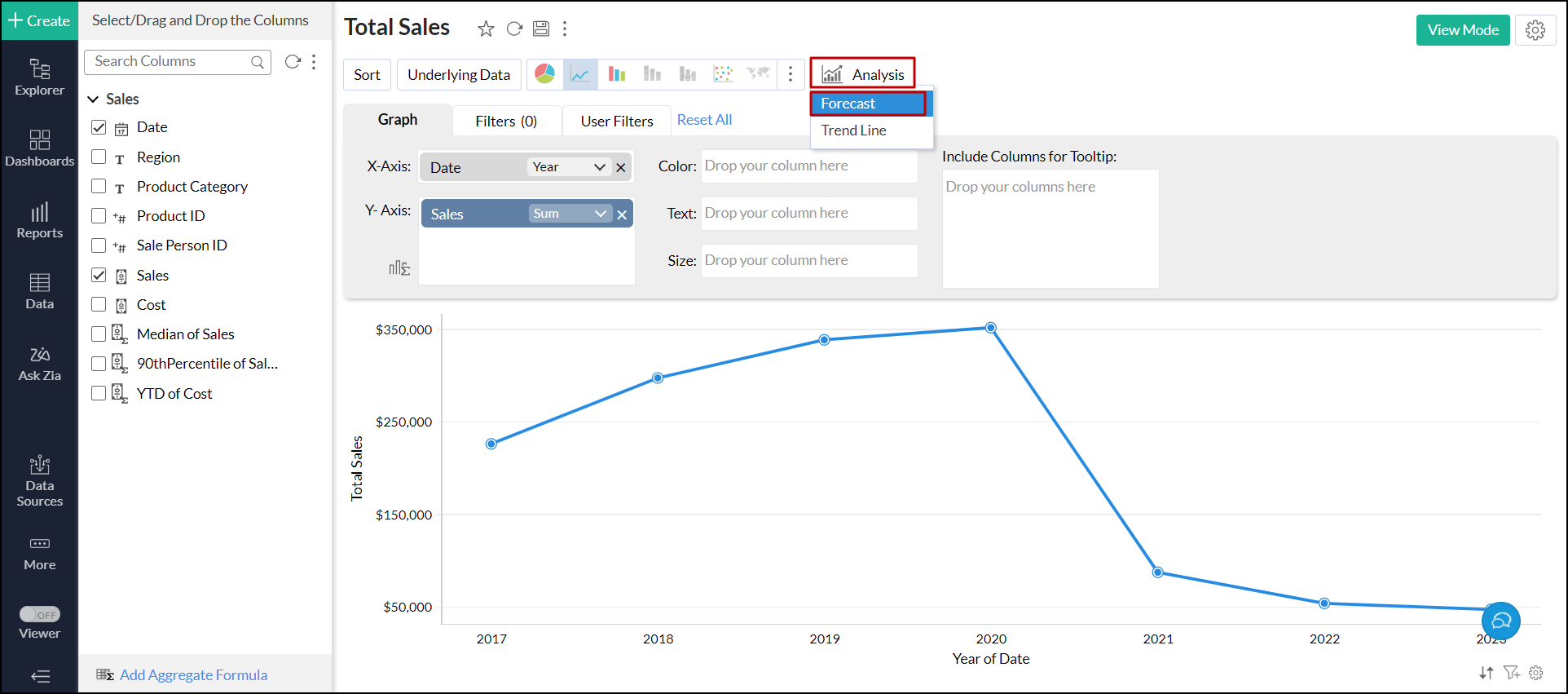• In the dialog that appears, click the + Add Forecast button and choose the Y-axis data that has to be forecasted.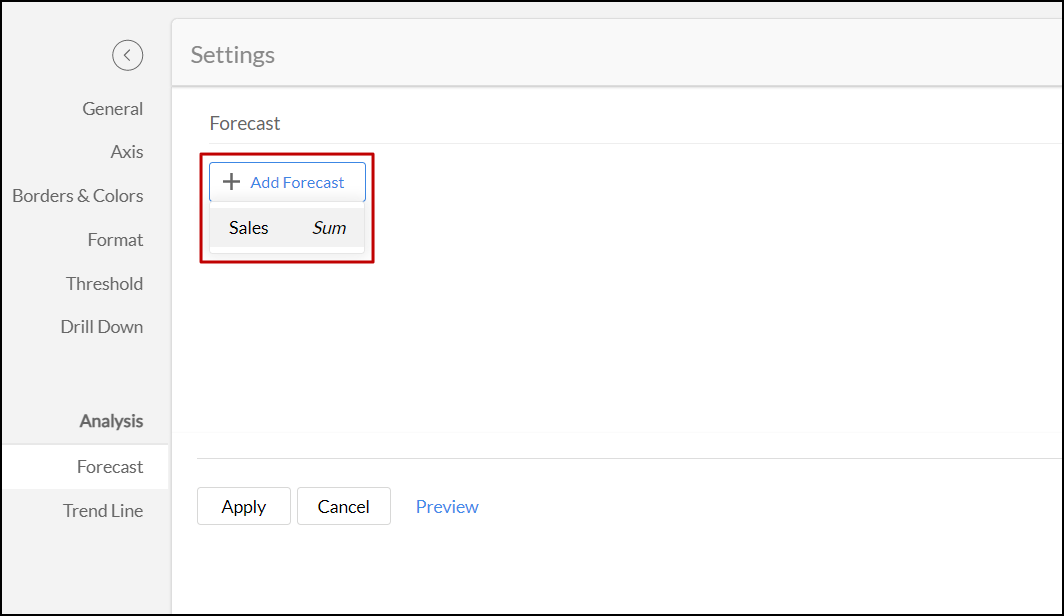• Choose the required model from the drop-down, either Auto or Custom.
• Auto: This option enables the forecast engine to choose the appropriate model automatically based on the given data.
• Custom: This option allows you to choose the required model to forecast the data. The following are the supported models: ETS, STL, ARIMA, and Regression. Click the corresponding links to learn about each model in detail.
• Specify the number of data series you need to forecast in the Forecast Length field.
• In the Ignore Last field, provide the number of data series that have to be ignored while forecasting the data.
• Choose the confidence interval in which the data point is likely to occur from the Confidence Percentage drop-down. Please note that this option applies only to the line chart.
• Provide a title for the forecasted series in the Legend Name field.
• Choose the required color and the line format for forecasting the data in the Formatting drop-down. If you have chosen Confidence Percentage, you also select the required style for it from the Formatting drop-down.
• Select the following options as required:
• Display Negative Forecasts as 0: Select this option to display all the negative values that have been forecasted as zero.
• Show Forecast Legend: This option allows you to add a legend for the forecasted data.
• Show Forecast Info: This option lets you add an option to view the forecast model applied over the chart.
• Show Ignored Values: This option enables you to include the data points that are ignored for forecasting.
• Click Apply to forecast the data.
• Click the Preview link to view the forecasted data before the actual forecasting is done.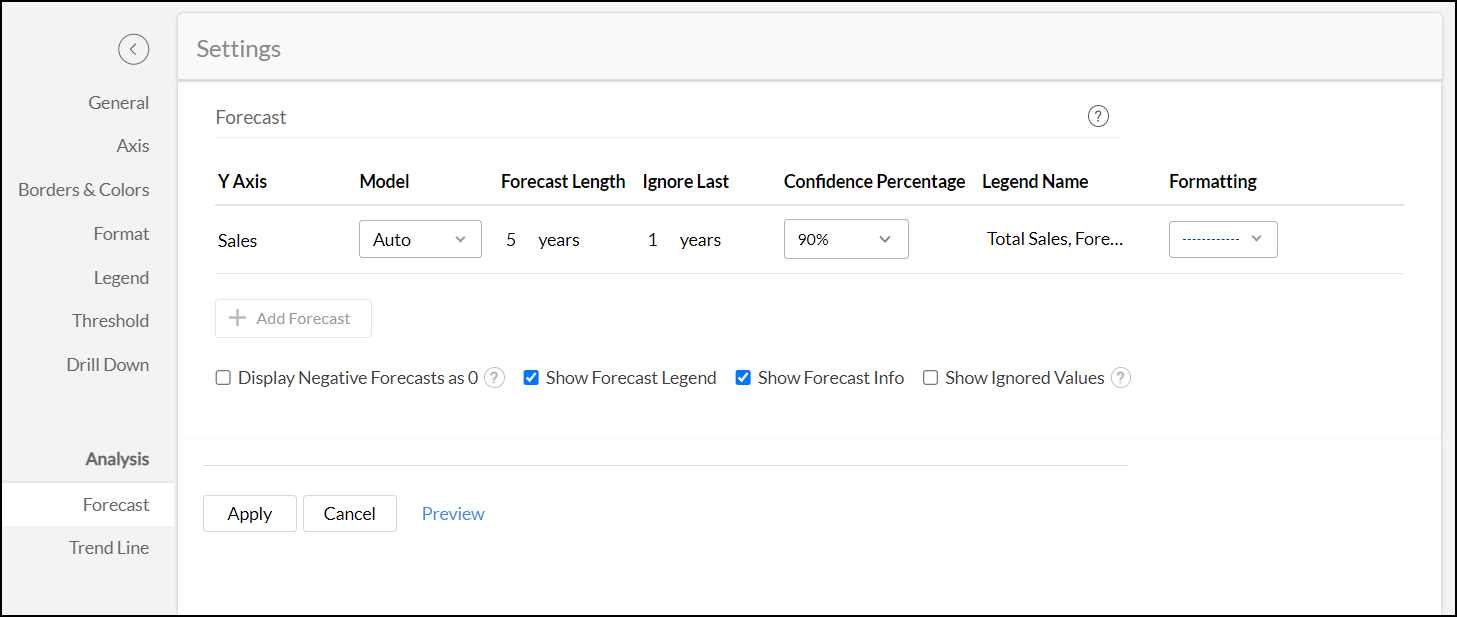The following is the line chart forecasted with a 90% confidence score.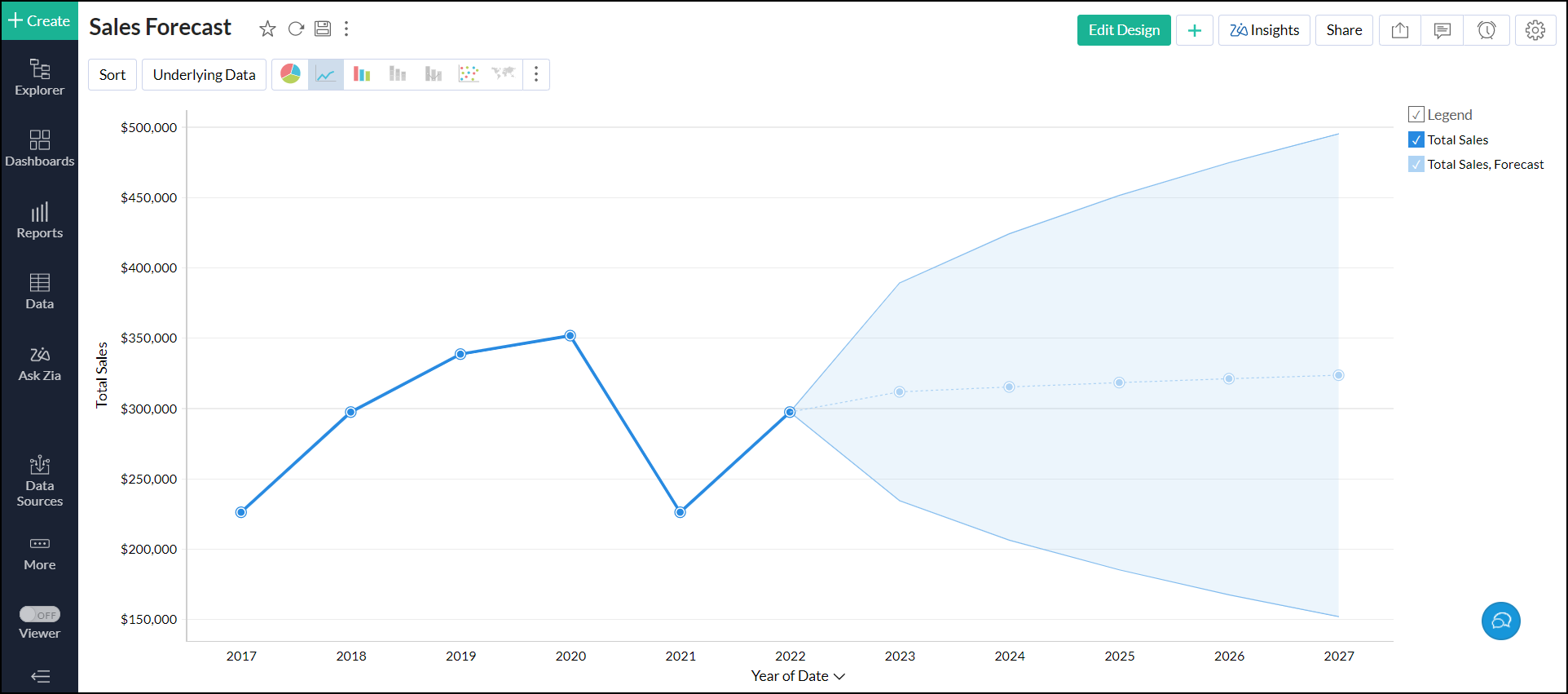### 4. Can I set up forecasting over multiple Y-Axis?

Yes. Analytics Plus allows you to set up forecasting over multiple Y-axis in charts.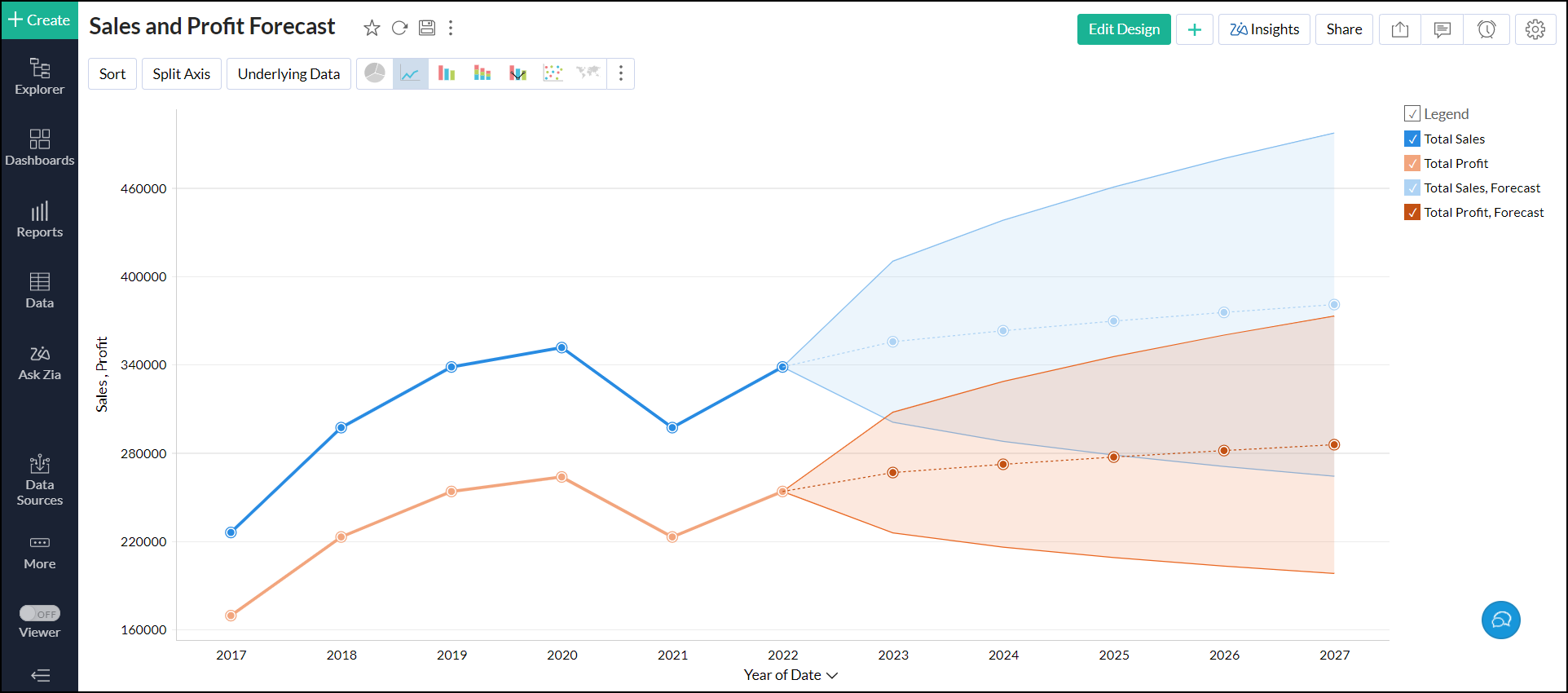### 5. Can I set up forecasting over a chart with colors?

Yes. Analytics Plus enables you to set up forecasting in charts that have columns on the Color shelf. To do this, apply forecasting based on Y-axis to forecast all the color series.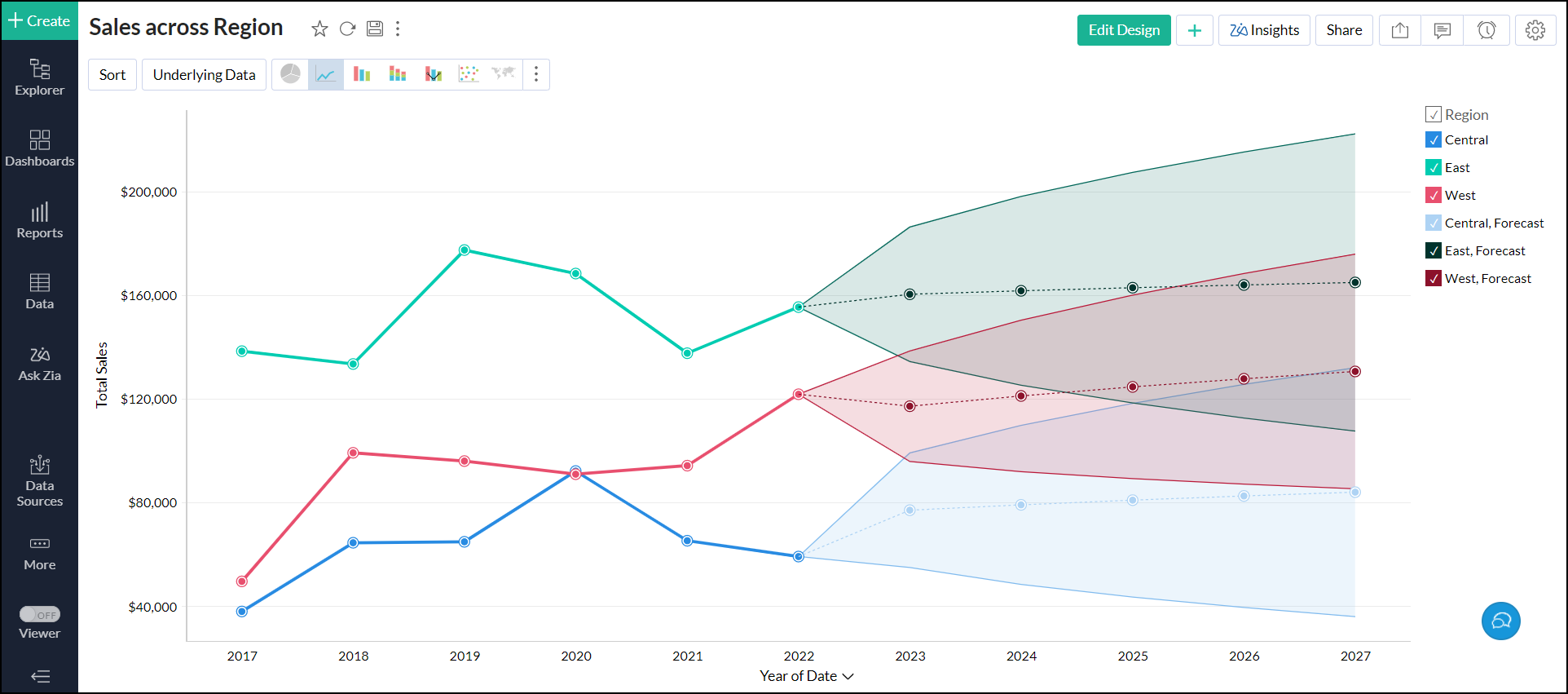### 6. How does forecasting work in Analytics Plus?

Analytics Plus offers a powerful forecasting engine that predicts future data points based on past data. The forecasting engine offers a range of customization such as the number of units to be forecasted, the number of data points to be ignored in the past data and the formatting to be applied over the forecasted data points.

The following points detail how forecasting works in Analytics Plus:

• The forecasting engine analyses past data points and identifies periodicity using autocorrelation techniques.
• The seasonality, trend, and randomness of the data are computed, which is then fine-tuned using iterative processing.
• The forecasting engine then runs linear, logarithmic, and exponential regressions to categorize the data series as linear, logarithmic, or exponential.
• The accuracy of these predictions is verified using hindcasting. This is a type of backtest that uses the prediction results to estimate past data points and verifies it with the actual data from the past.
• Once the verification is complete, the forecasting engine displays the forecasted data points.

### 7. Why are the forecasted points shown differently for the shared users?

If you have shared the chart with different filter criteria to users, then the number of past data points will vary for different users. Hence the forecasted points are also different for shared users.

### 8. Is the underlying data option available for forecasted data points?

No. Analytics Plus does not generate any underlying data for the forecasted data points. So the View Underlying Data and Drill Down options are not able for the forecasted data points.

### 9. Can I view how forecasting is applied to the data?

Yes. Analytics Plus lets you view the type of forecast model applied over the charts. To view the forecast information, follow the below steps.

In View mode, click any one of the forecasted data points.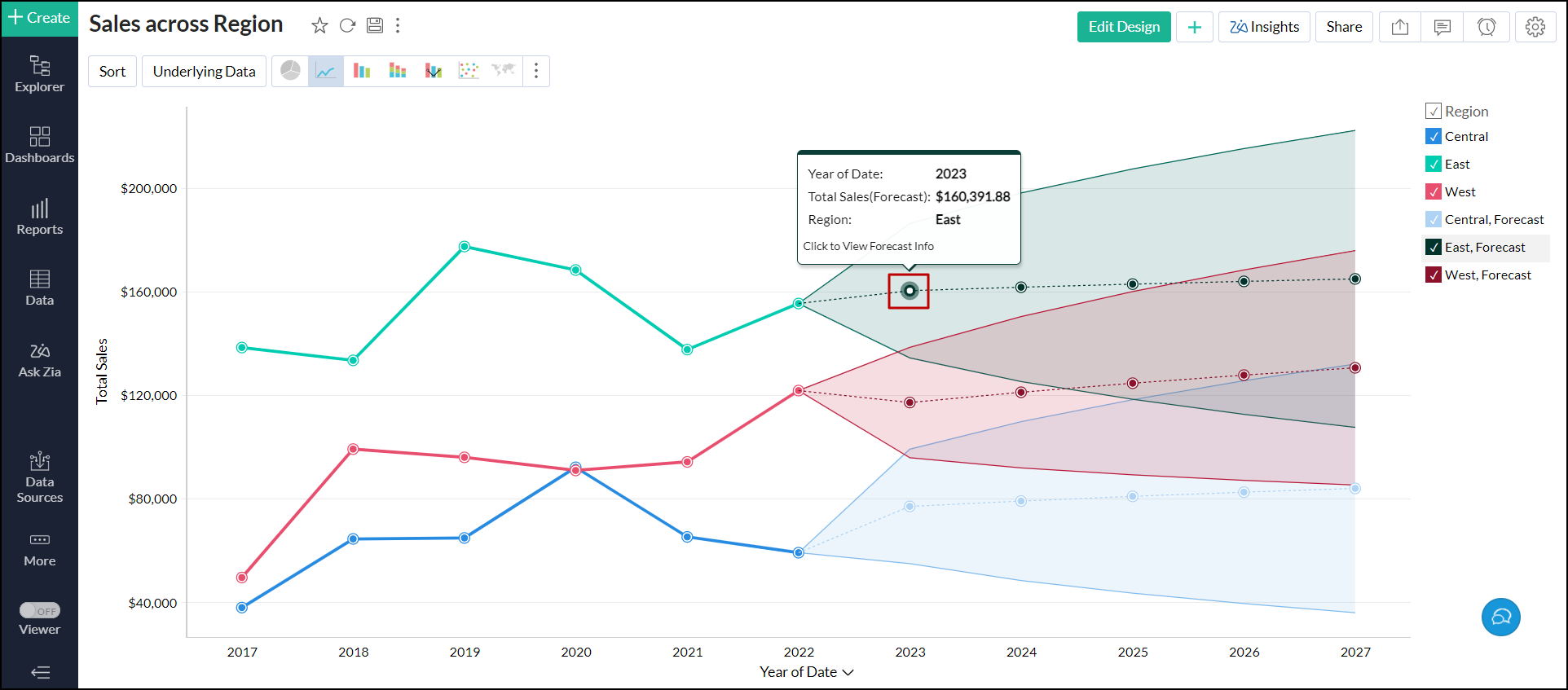You also navigate to the chart's Edit Design mode, click the Settings icon > Forecast tab, and click the Forecast Info icon that appears on mouse over the required forecast.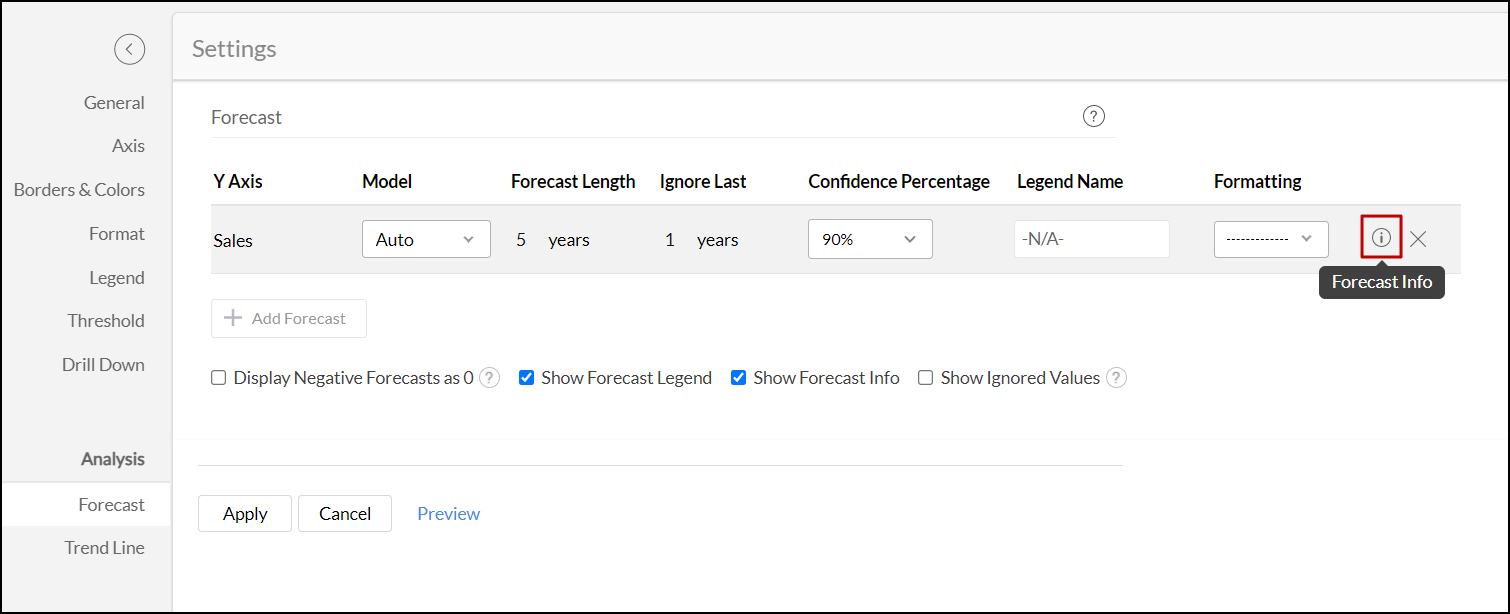The forecast model dialog appears with the following information:

#### General information

This section displays the following information:

• Training Period: Displays the period of actual data based on which forecast is applied.
• Forecast Period: Displays the forecast period.

#### Model information

This section displays the type of forecast model applied over the chart.

 Field Description Common Fields Forecast Model Displays the name of the forecast model applied over the chart. {Forecast Model} Model Displays the name of the sub-model applied over the chart. Frequency Gives the frequency of the time series. Data Components Displays whether trend and seasonality are present in the given data. ARIMA Arima Coefficients Gives the weight of past observations of the data. This will decay or go to zero when the current observation is more closely related to the most recent observations rather than to old observations. Note: This value will be hidden when there are no autoRegressive, movingAverage, seasonalAutoRegressive & seasonalMovingAverage terms. i,e., when p=q=P=Q=0 STL Decomposed Values Splits the actual data and displays it as three components namely Trend, Seasonality, and Residue. ETS Shows the smoothing parameters for Level, Trend, and Seasonality. Regression R Square This value indicates how better the model fits the actual data. Adjusted R Squared This value ensures that the model does not overfit. The model with the highest Adjusted R Squared will be applied to the data. F Statistic This value shows how far the X value influences the trend. The higher the value, the more it (X value) contributes to the trend. P Value This value shows the probability of the estimator not contributing to the data (significance of the Coefficients). The smaller the p-value, the more significant the model is.

#### Performance indicator

The performance indicator section shows the following information:

• RMSE: The Root Mean Square Error shows how concentrated the data is around a trend.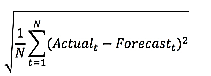• MAPE: The Mean Absolute Percentage Error is a measure of prediction accuracy of a forecasting method in statistics.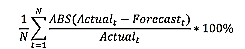• WMAPE: It's a variant of MAPE in which errors are weighted by values of actuals.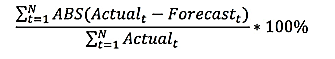• Quality: The Forecast quality is calculated based on LEP. The possible values are:
• GOOD
• ACCEPTABLE
• POOR
• AICC: This is a model to check the quality of the forecast.• BIC: This is a model to check the quality of the forecast. Following is the formula.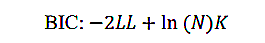Where,

LL - The model log likelihood estimate

K - Number of parameters (e.g., 3 if (alpha, beta & gamma) are used)

n - Sample size

• LEPS: Linear Error in Probability Space is the mean absolute difference between the forecast cumulative distribution value (CDF) and the observation.

## Troubleshooting Tips

### 1. Forecasting is disabled as the data is completely ignored.

This error occurs when the ignore all the past data points from Ignore Last setting is enabled. Disable the setting to resolve the error.

### 2. Forecasting is disabled as there is not enough data to identify pattern.

This error occurs when there is no sufficient data produced to the forecasting engine to come up with forecasted data points.

### 3. Forecasting is disabled as there are more than 40% empty values.

This error occurs when the given data points have more null values. Null values might lead to inaccurate forecasting of data, so the forecasting engine discards the process when the null values are more than 40% in the given data.

### 4. Column cannot be forecast as more than 5 data points is required.

This error occurs if the data points to be considered for forecasting are less than 5 points. To produce an accurate forecast, the chart should contain more than 5 data points.